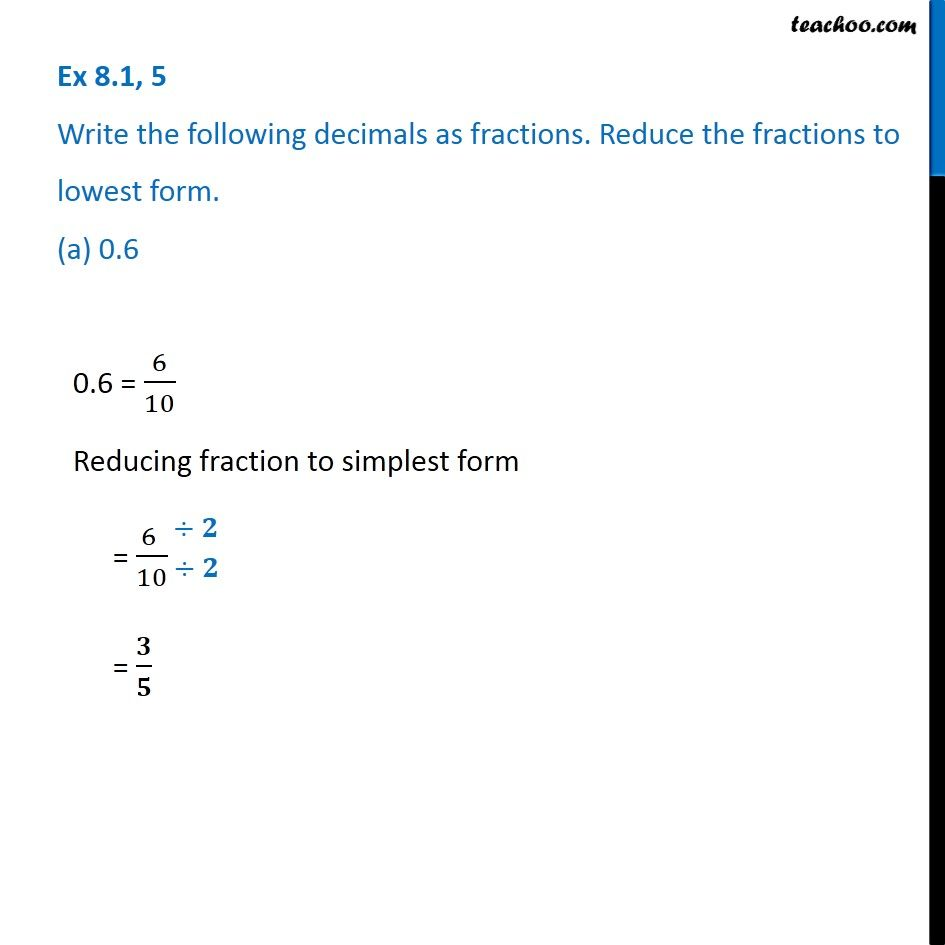1. Chapter 8 Class 6 Decimals
2. Concept wise
3. Decimals to fractions

Transcript

Ex 8.1, 5 Write the following decimals as fractions. Reduce the fractions to lowest form. (a) 0.6 0.6 = 6/10 Reducing fraction to simplest form = (6 )/10 = 𝟑/𝟓